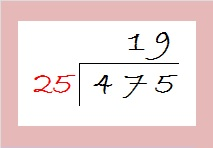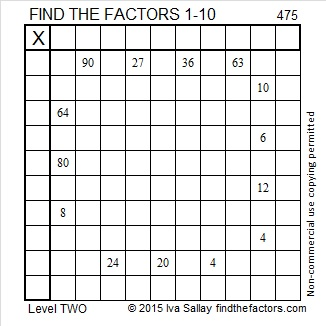# 475 and Level 2

Numbers ending in 25, 50, 75, and 00 can be evenly divided by 25 so their square roots can be simplified. What is 475 divided by 25? You can find the answer by asking yourself, “If I had \$4.75 in quarters, how many quarters would I have?”

If you had \$5.00 in quarters, you would have 20 quarters, so \$4.75 is 19 quarters. Thus √475 = (√25)(√19) = 5√19.What is the greatest common factor of the numbers in this Pythagorean triple, 133-456-475? Let’s look at the difference between 456 and the other two numbers:

• 456 – 133 = 323
• 475 – 456 = 19
• Since 19 is the smaller difference, the greatest common factor has to be a factor of 19, and 19 just happens to be a factor of all three numbers.
• 7-24-25 multiplied by 19 is 133-456-475.

What is the greatest common factor of the numbers in Pythagorean triple, 285-380-475? Find the difference between 380 and the other two numbers:

• 380 – 285 = 95
• 475 – 380 = 95
• Since the differences are equal, we know that this Pythagorean triple is just 3-4-5 multiplied by 95, and the greatest common factor is 95.

Here is today’s factoring puzzle:Print the puzzles or type the solution on this excel file: 10 Factors 2015-04-27

—————————————————————————————————

• 475 is a composite number.
• Prime factorization: 475 = 5 x 5 x 19, which can be written 475 = (5^2) x 19
• The exponents in the prime factorization are 2 and 1. Adding one to each and multiplying we get (2 + 1)(1 + 1) = 3 x 2  = 6. Therefore 475 has exactly 6 factors.
• Factors of 475: 1, 5, 19, 25, 95, 475
• Factor pairs: 475 = 1 x 475, 5 x 95, or 19 x 25
• Taking the factor pair with the largest square number factor, we get √475 = (√25)(√19) = 5√19 ≈ 21.79449—————————————————————————————————This site uses Akismet to reduce spam. Learn how your comment data is processed.